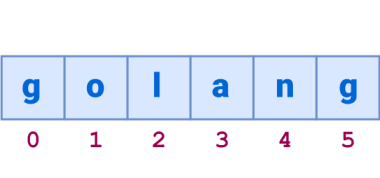golang arrays can hold multiple values. The minimum elements of an array is zero, but they usually have two or more. Each element in an array has a unique index.

The index starts at zero (0). That means to access the first element of an array, you need to use the zeroth index.

Related course: Introducing Go: Build Reliable, Scalable Programs

## Arrays in golang

### Introduction to arrays

An array is a set of numbered and length-fixed data item sequences that have the same unique type

This type can be any primitive type such as:

• integer
• string
• custom type

The array length must be a constant expression and must be a non-negative integer.

An array in the Go language is a type of value (not a pointer to the first element in C/C++), so it can be created by `new()`:

The array element can be read (or modified) by an index (position), the index starts from 0, the first element index is 0, the second index is 1, and so on.

Note The array starts at 0 in many programming languages, including Golang, C++, C, C# and PythonThe number of elements, also known as length or array size, must be fixed and given when the array is declared (need to know the array length to allocate memory at compile time); the array length is up to 2 Gb.

If each element is an integer value, all elements are automatically initialized to the default value of 0 when the array is declared.

The method of traversing an array can either be a condition loop or a for-range can be used.Both of these structures are equally applicable to Slices.

Here is an array of non-fixed length that is determined by the number of elements specified at initialization time.

Several assignment modes:

### Example

The program below is an example of an array loop in golang.
The array we define has several elements. We print the first item with the zeroth index.

Upon running this program it will output the first (1) and second (2) element of the array. They are referenced by the zeroth and first index. In short: computers start counting from 0.

This program will output:

The index should not be larger than the array, that could throw an error or unexpected results.

Video below

### Exercises

1. Create an array with the number 0 to 10
2. Create an array of strings with names